# [Week 12] NPTEL Introduction To Machine Learning Assignment Answer 2023NPTEL Introduction To Machine Learning Assignment Answer

## NPTEL Introduction To Machine Learning Week 12 Assignment Answer 2023

Q1. You want to make an RL agent for a game where 2 players compete to win (like Chess and Go). Which among the given would be the best approach for this?
Play against best human players
Iteratively play against the best (fixed) version of itself
Play against a supervised agent trained on demonstrations of best human players
Watch thousands of games being played and learn the patterns in an unsupervised manner

`Answer:- b`

Q2. Statement 1: Empirical error is always greater than generalisation error.
Statement 2: Training data and test data have different underlying(true) distributions.
Choose the correct option:
Statement 1 is true. Statement 2 is true. Statement 2 is the correct reason for statement 1.
Statement 1 is true. Statement 2 is true. Statement 2 is not the correct reason for statement 1.
Statement 1 is true. Statement 2 is false.
Both statements are false.

[ihc-hide-content ihc_mb_type=”show” ihc_mb_who=”1,2,3″ ihc_mb_template=”1″ ]

`Answer:- c`

Q3.

`Answer:- d`

Q4.

`Answer:- c`

Q5. Statement A: Reinforcement learning is a type of unsupervised learning.
Statement B: Reinforcement learning does not have labels.
Both statements are true. Statement B is the correct explanation for statement A.
Both statements are true. Statement B is NOT the correct explanation for statement A.
Statement A is true. Statement B is false.
Statement A is false. Statement B is true.
Both statements are false.

`Answer:- e`

Q6. What is a policy in reinforcement learning?
A mapping from states to actions
A mapping from states to rewards
A mapping from actions to rewards
A mapping from actions to next state

`Answer:- a`

Q7.

`Answer:- d`

[/ihc-hide-content]

## NPTEL Introduction To Machine Learning Week 11 Assignment Answer 2023

Q1. What is the update for πk in EM algorithm for GMM?

`Answer:- a`

Q2. Consider the two statements:
Statement 1: The EM algorithm can only be used for parameter estimation of mixture models.
Statement 2: The Gaussian Mixture Models used for clustering always outperform k-means and single-link clustering.
Which of these are true?
Both the statements are true
Statement 1 is true, and Statement 2 is false
Statement 1 is false, and Statement 2 is true
Both the statements are false

`Answer:- d`

Q3. KNN is a special case of GMM with the following properties: (Select all that apply)

`Answer:- b, d`

Q4. What does soft clustering mean in GMMs?
There may be samples that are outside of any cluster boundary.
The updates during maximum likelihood are taken in small steps, to guarantee convergence.
It restricts the underlying distribution to be gaussian.
Samples are assigned probabilities of belonging to a cluster.

`Answer:- d`

Q5. In Gaussian Mixture Models, πi
are the mixing coefficients. Select the correct conditions that the mixing coefficients need to satisfy for a valid GMM model.

`Answer:- a, c`

Q6. What statement(s) are true about the expectation-maximization (EM) algorithm?

• It requires some assumption about the underlying probability distribution.
• Comparing to a gradient descent algorithm that optimizes the same objective function as EM, EM may only find a local optima, whereas the gradient descent will always find the global optima
• The EM algorithm minimizes a lower bound of the marginal likelihood P(D;θ)
• The algorithm assumes that some of the data generated by the probability distribution are not observed.
`Answer:- a, d`

Q7. Consider the two statements:
Statement 1: The EM algorithm can get stuck at saddle points.
Statement 2: EM is guaranteed to converge to a point with zero gradient.

Which of these are true?

Both the statements are true
Statement 1 is true, and Statement 2 is false
Statement 1 is false, and Statement 2 is true
Both the statements are false

`Answer:- a`

## NPTEL Introduction To Machine Learning Week 10 Assignment Answer 2023

1. The pairwise distance between 6 points is given below. Which of the option shows the hierarchy of clusters created by single link clustering algorithm?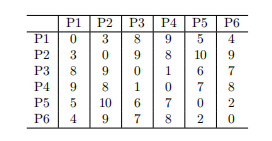`Answer :- b`

2. For the pairwise distance matrix given in the previous question, which of the following shows the hierarchy of clusters created by the complete link clustering algorithm.

`Answer :- b`
`Answer :- c`

4. Run K-means on the input features of the MNIST dataset using the following initialization:

K Means(nclusters=10,randomstate=seed)

Usually, for clustering tasks, we are not given labels, but since we do have labels for our dataset, we can use accuracy to determine how good our clusters are.

Label the prediction class for all the points in a cluster as the majority true label. E.g. {a,a,b} would be labeled as {a,a,a}

What is the accuracy of the resulting labels?
0.790
0.893
0.702
0.933

`Answer :- a`
`Answer :- d`

6. a in rand-index can be viewed as true positives(pair of points belonging to the same cluster) and
b as true negatives(pair of points belonging to different clusters). How, then, are rand-index and accuracy from the previous two questions related?

• rand-index = accuracy
• rand-index = 1.18×accuracy
• rand-index = accuracy/2
• None of the above
`Answer :- d`

7. Run BIRCH on the input features of MNIST dataset using Birch(nclusters=10,threshold=1). What is the rand-index obtained?

• 0.91
• 0.96
• 0.88
• 0.98
`Answer :- b`

8. Run PCA on MNIST dataset input features with n components = 2. Now run DBSCAN using DBSCAN(eps=0.5,minsamples=5) on both the original features and the PCA features. What are their respective number of outliers/noisy points detected by DBSCAN?

As an extra, you can plot the PCA features on a 2D plot using matplotlib.pyplot.scatter with parameter c=y−pred (where y−pred is the cluster prediction) to visualise the clusters and outliers.

• 1600, 1522
• 1500, 1482
• 1000, 1000
• 1797, 1742
`Answer :- d`

[/ihc-hide-content]

## NPTEL Introduction To Machine Learning Week 9 Assignment Answer 2023

1. Which of the following best describes the Markov property in a Hidden Markov Model (HMM)?

• The future state depends on the current state and the entire past sequence of states.
• The future state depends only on the current state and is independent of the past states, given the current state.
• The future state depends on the past states and the future states, given the current state.
• The future state depends only on the past states and is independent of the current state.
`Answer :- b`

2. Statement 1: Probability distributions are valid potential functions.
Statement 2: Probability is always strictly positive.

• Statement 1 is true. Statement 2 is true. Statement 2 is the correct reason for statement 1.
• Statement 1 is true. Statement 2 is true. Statement 2 is not the correct reason for statement 1.
• Statement 1 is true. Statement 2 is false.
• Both statements are false.
`Answer :- c`

3. In the undirected graph given below, which nodes are conditionally independent of each other given B? Select all that apply.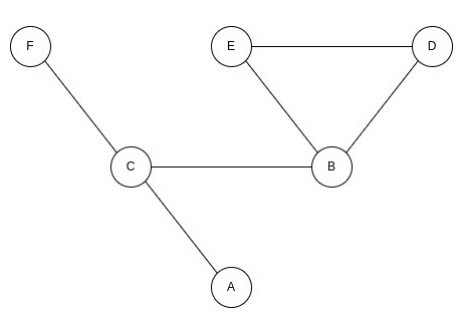• C, D
• D, E
• E, C
• A, F
• None of the above
`Answer :- a, c`

4. Given graph below:

Factorization is:

p(x,y,z)=p(x)p(y|x)p(y|z)

p(x,y,z)=p(y)p(x|y)p(z|y)

p(x,y,z)=p(z)p(z|y)p(x|y)

p(x,y,z)=p(y)p(y|x)p(y|z)

`Answer :- b`

5. For the given graphical model, what is the optimal variable elimination order when trying to calculate P(E=e)?

• A, B, C, D
• D, C, B, A
• A, D, B, C
• D, A, C, A
`Answer :- a`

6. Which of the following methods are used for calculating conditional probabilities? (more than one may apply)

• Viterbi algorithm
• MAP inference
• Variable elimination
• Belief propagation
`Answer :- b, d`

7. In the undirected graph given below, which nodes are conditionally independent of each other given a single other node (may be different for different pairs)? Select all that apply.

• 3, 2
• 0, 4
• 2, 5
• 1, 5
`Answer :- a, d `

## NPTEL Introduction To Machine Learning Week 8 Assignment Answer 2023

1. The figure below shows a Bayesian Network with 9 variables, all of which are binary.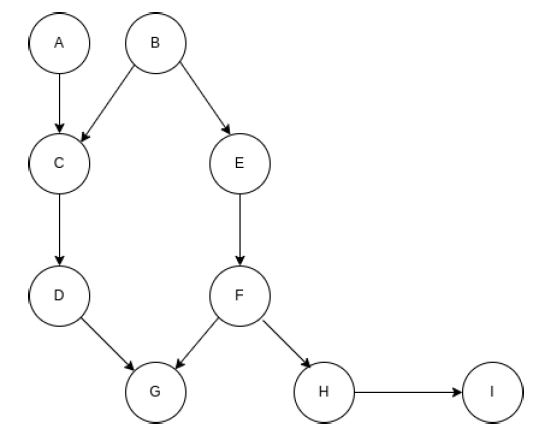Which of the following is/are always true for the above Bayesian Network?

• P(A,B|G)=P(A|G)P(B|G)
• P(A,I)=P(A)P(I)
• P(B,H|E,G)=P(B|E,G)P(H|E,G)
• P(C|B,F)=P(C|F)
`Answer :- b `

2. Consider the following data for 20 budget phones, 30 mid-range phones, and 20 high-end phones:

Consider a phone with 2 SIM card slots and NFC but no 5G compatibility. Calculate the probabilities of this phone being a budget phone, a mid-range phone, and a high-end phone using the Naive Bayes method. The correct ordering of the phone type from the highest to the lowest probability is?

• Budget, Mid-Range, High End
• Budget, High End, Mid-Range
• Mid-Range, High End, Budget
• High End, Mid-Range, Budget
`Answer :- c`

3. A dataset with two classes is plotted below.

Does the data satisfy the Naive Bayes assumption?

• Yes
• No
• The given data is insufficient
• None of these
`Answer :- b`

4. A company hires you to look at their classification system for whether a given customer would potentially buy their product. When you check the existing classifier on different folds of the training set, you find that it manages a low accuracy of usually around 60%. Sometimes, it’s barely above 50%. With this information in mind, and without using additional classifiers, which of the following ensemble methods would you use to increase the classification accuracy effectively?

• Committee Machine
• Bagging
• Stacking
`Answer :- b`

5. Which of the following algorithms don’t use learning rate as a hyperparameter?

• Random Forests
• KNN
• PCA
`Answer :- a, c, d`

6. Consider the two statements:
Statement 1:
Bayesian Networks need not always be Directed Acyclic Graphs (DAGs)
Statement 2: Each node in a bayesian network represents a random variable, and each edge represents conditional dependence.
Which of these are true?

• Both the statements are True.
• Statement 1 is true, and statement 2 is false.
• Statement 1 is false, and statement 2 is true.
• Both the statements are false.
`Answer :- c`

7. A dataset with two classes is plotted below.

Does the data satisfy the Naive Bayes assumption?

• Yes
• No
• The given data is insufficient
• None of these
`Answer :- a`

8. Consider the below dataset:

Suppose you have to classify a test example “The ball won the race to the boundary” and are asked to compute P(Cricket |“The ball won the race to the boundary”), what is an issue that you will face if you are using Naive Bayes Classifier, and how will you work around it? Assume you are using word frequencies to estimate all the probabilities.

• There won’t be a problem, and the probability of P(Cricket |“The ball won the race to the boundary”) will be equal to 1.
• Problem: A few words that appear at test time do not appear in the dataset.
• Solution: Smoothing.
• Problem: A few words that appear at test time appear more than once in the dataset.
• Solution: Remove those words from the dataset.
• None of these
`Answer :- b`

## NPTEL Introduction To Machine Learning Week 7 Assignment Answer 2023

1. What is bootstrapping in the context of machine learning?

• A technique to improve model training speed.
• A method to reduce the size of the dataset.
• Creating multiple datasets by randomly sampling with replacement.
• A preprocessing step to normalize data.
`Answer :- c`

2. Which of the following is NOT a benefit of cross-validation?

• Reduces the risk of overfitting.
• Provides a more accurate estimate of model performance.
• Allows for better understanding of model bias.
• Increases the size of the training dataset.
`Answer :- d`

3. Bagging is an ensemble method that:

• Focuses on boosting the performance of a single weak learner.
• Trains multiple models sequentially, each learning from the mistakes of the previous one.
• Combines predictions of multiple models to improve overall accuracy.
• Utilizes a committee of diverse models for prediction.
`Answer :- c`

4. Which evaluation measure is more suitable for imbalanced classification problems?

• Accuracy
• Precision
• F1-score
• Mean Squared Error
`Answer :- c`

5. What does the ROC curve represent?

• The trade-off between precision and recall.
• The relationship between accuracy and F1-score.
• The performance of a model across various thresholds.
• The distribution of classes in a dataset.
`Answer :- c`

6. Which ensemble method involves training multiple models in such a way that each model corrects the errors of the previous model?

• Bagging
• Stacking
• Boosting
• Committee Machines
`Answer :- c`

7. In a ROC curve, what does the diagonal line represent?

• The perfect classifier
• Random guessing
• Trade-off between sensitivity and specificity
• The ideal threshold for classification
`Answer :- b`

8. In k-fold cross-validation, how is the dataset divided for training and testing?

• The dataset is randomly shuffled and divided into k equal parts. One part is used for testing and the remaining k-1 parts are used for training.
• The dataset is split into two equal parts: one for training and the other for testing.
• The dataset is divided into k equal parts. One part is used for testing and the remaining k-1 parts are used for training in each iteration.
• The dataset is divided into k unequal parts based on data distribution.
`Answer :- c`

9. What is the primary advantage of ensemble methods over individual models?

• Simplicity of implementation
• Lower computational complexity
• Increased Robustness
• Faster training time
`Answer :- c`

## NPTEL Introduction To Machine Learning Week 6 Assignment Answer 2023

1. Which of the following is/are major advantages of decision trees over other supervised learning techniques? (Note that more than one choices may be correct)

• Theoretical guarantees of performance
• Higher performance
• Interpretability of classifier
• More powerful in its ability to represent complex functions
`Answer :- c`

2. Increasing the pruning strength in a decision tree by reducing the maximum depth:

• Will always result in improved validation accuracy.
• Will lead to more overfitting.
• Might lead to underfitting if set too aggressively.
• Will have no impact on the tree’s performance.
• Will eliminate the need for validation data.
`Answer :- c`

3. Consider the following statements:
Statement 1:
Decision Trees are linear non-parametric models.
Statement 2: A decision tree may be used to explain the complex function learned by a neural network.

Both the statements are True.
Statement 1 is True, but Statement 2 is False.
Statement 1 is False, but Statement 2 is True.
Both the statements are False.

`Answer :- c`

4. Consider the following dataset:

What is the initial entropy of Malignant?

• 0.543
• 0.9798
• 0.8732
• 1
`Answer :- b`

5. For the same dataset, what is the info gain of Vaccination?

• 0.4763
• 0.2102
• 0.1134
• 0.9355
`Answer :- a`

6. Which of the following machine learning models can solve the XOR problem without any transformations on the input space?

• Linear Perceptron
• Neural Networks
• Decision Trees
• Logistic Regression
`Answer :- b, c`

7. Statement: Decision Tree is an unsupervised learning algorithm.
Reason: The splitting criterion use only the features of the data to calculate their respective measures

• Statement is True. Reason is True.
• Statement is True. Reason is False.
• Statement is False. Reason is True.
• Statement is False. Reason is False.
`Answer :- d`

8. ______ is a measurement of likelihood of an incorrect classification of a new instance for a random variable, if the new instance is randomly classified as per the distribution of class labels from the data set.

• Gini impurity.
• Entropy.
• Information gain.
• None of the above.
`Answer :- a`

9. What is a common indicator of overfitting in a decision tree?

• The training accuracy is high while the validation accuracy is low.
• The tree is shallow.
• The tree has only a few leaf nodes.
• The tree’s depth matches the number of attributes in the dataset.
• The tree’s predictions are consistently biased.
`Answer :- a`

10. Consider a dataset with only one attribute(categorical). Suppose, there are 10 unordered values in this attribute, how many possible combinations are needed to find the best split-point for building the decision tree classifier? (considering only binary splits)

• 10
• 511
• 1023
• 512
`Answer :- b`

## NPTEL Introduction To Machine Learning Week 5 Assignment Answer 2023

1. The perceptron learning algorithm is primarily designed for:

2. Unsupervised learning
`Answer :- d`

2. The last layer of ANN is linear for and softmax for .

• Regression, Regression
• Classification, Classification
• Regression, Classification
• Classification, Regression
`Answer :- c`

3. Consider the following statement and answer True/False with corresponding reason:
The class outputs of a classification problem with a ANN cannot be treated independently.

1. True. Due to cross-entropy loss function
2. True. Due to softmax activation
3. False. This is the case for regression with single output
4. False. This is the case for regression with multiple outputs
`Answer :- b`

4. Given below is a simple ANN with 2 inputs X1,X2∈{0,1} and edge weights −3,+2,+2

Which of the following logical functions does it compute?

1. XOR
2. NOR
3. NAND
4. AND
`Answer :- d`

5. Using the notations used in class, evaluate the value of the neural network with a 3-3-1 architecture (2-dimensional input with 1 node for the bias term in both the layers). The parameters are as follows

Using sigmoid function as the activation functions at both the layers, the output of the network for an input of (0.8, 0.7) will be (up to 4 decimal places)

1. 0.7275
2. 0.0217
3. 0.2958
4. 0.8213
5. 0.7291
6. 0.8414
7. 0.1760
8. 0.7552
9. 0.9442
10. None of these
`Answer :- f`

6. If the step size in gradient descent is too large, what can happen?

1. Overfitting
2. The model will not converge
3. We can reach maxima instead of minima
4. None of the above
`Answer :- b`

7. On different initializations of your neural network, you get significantly different values of loss. What could be the reason for this?

1. Overfitting
2. Some problem in the architecture
3. Incorrect activation function
4. Multiple local minima
`Answer :- d`

8. The likelihood L(θ|X) is given by:

1. P(θ|X)
2. P(X|θ)
3. P(X).P(θ)
4. P(θ)P(X)
`Answer :- b`

9. Why is proper initialization of neural network weights important?

1. To ensure faster convergence during training
2. To prevent overfitting
3. To increase the model’s capacity
4. Initialization doesn’t significantly affect network performance
5. To minimize the number of layers in the network
`Answer :- a`

10. Which of these are limitations of the backpropagation algorithm?

1. It requires error function to be differentiable
2. It requires activation function to be differentiable
3. The ith layer cannot be updated before the update of layer i+1 is complete
4. All of the above
5. (a) and (b) only
6. None of these
`Answer :- d`

## NPTEL Introduction To Machine Learning Week 4 Assignment Answer 2023

Q1. Consider the data set given below.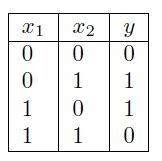Claim: PLA (perceptron learning algorithm) can learn a classifier that achieves zero misclassification error on the training data. This claim is:

True
False
Depends on the initial weights
True, only if we normalize the feature vectors before applying PLA.

`Answer:- b`

Q2. Which of the following loss functions are convex? (Multiple options may be correct)

• 0-1 loss (sometimes referred as mis-classification loss)
• Hinge loss
• Logistic loss
• Squared error loss
`Answer:- b, c, d`

Q3. Which of the following are valid kernel functions?

• (1+ < x, x’ >)d
• tanℎ(K1<x,x’>+K2)
• exp(−γ||x−x’||2)
`Answer:- a, b, c`

Q4. Consider the 1 dimensional dataset: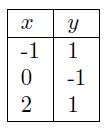(Note: x is the feature, and y is the output)

State true or false: The dataset becomes linearly separable after using basis expansion with the following basis function ϕ(x)=[1x3]

• True
• False
`Answer:- b`

Q5. State True or False:
SVM cannot classify data that is not linearly separable even if we transform it to a higherdimensional space.

• True
• False
`Answer:- b`

Q6. State True or False:
The decision boundary obtained using the perceptron algorithm does not depend on the initial values of the weights.

• True
• False
`Answer:- b`

Q7. Consider a linear SVM trained with n labeled points in R2 without slack penalties and resulting in k=2 support vectors, where n>100. By removing one labeled training point and retraining the SVM classifier, what is the maximum possible number of support vectors in the resulting solution?

• 1
• 2
• 3
• n − 1
• n
`Answer:- d`

Q8. Consider an SVM with a second order polynomial kernel. Kernel 1 maps each input data point x to K1(x)=[x x2]. Kernel 2 maps each input data point x to K2(x)=[3x 3×2]. Assume the hyper-parameters are fixed. Which of the following option is true?

• The margin obtained using K2(x) will be larger than the margin obtained using K1(x).
• The margin obtained using K2(x) will be smaller than the margin obtained using K1(x).
• The margin obtained using K2(x) will be the same as the margin obtained using K1(x).
`Answer:- a`

## NPTEL Introduction To Machine Learning Week 3 Assignment Answer 2023

1. Which of the following are differences between LDA and Logistic Regression?

• Logistic Regression is typically suited for binary classification, whereas LDA is directly applicable to multi-class problems
• Logistic Regression is robust to outliers whereas LDA is sensitive to outliers
• both (a) and (b)
• None of these
`Answer :- c`

2. We have two classes in our dataset. The two classes have the same mean but different variance.

LDA can classify them perfectly.
LDA can NOT classify them perfectly.
LDA is not applicable in data with these properties
Insufficient information

`Answer :- b`

3. We have two classes in our dataset. The two classes have the same variance but different mean.

LDA can classify them perfectly.
LDA can NOT classify them perfectly.
LDA is not applicable in data with these properties
Insufficient information

`Answer :- d`

4. Given the following distribution of data points:

What method would you choose to perform Dimensionality Reduction?

Linear Discriminant Analysis
Principal Component Analysis
Both LDA and/or PCA.
None of the above.

`Answer :- a`

5. If log(1−p(x)/1+p(x))=β0+βx What is p(x) ?

p(x)=1+eβ0+βx / eβ0+βx
p(x)=1+eβ0+βx / 1−eβ0+βx
p(x)=eβ0+βx / 1+eβ0+βx
p(x)=1−eβ0+βx / 1+eβ0+βx

`Answer :- d`

6. For the two classes ’+’ and ’-’ shown below.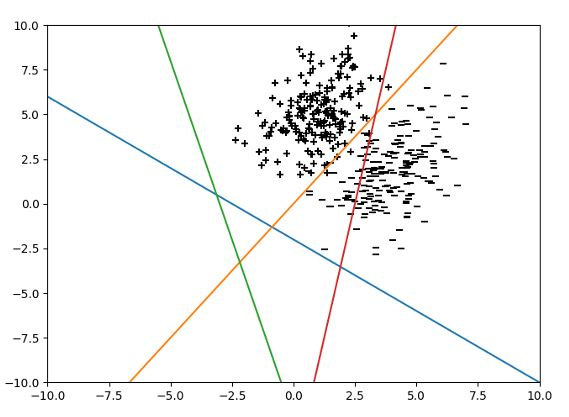While performing LDA on it, which line is the most appropriate for projecting data points?

Red
Orange
Blue
Green

`Answer :- c`

7. Which of these techniques do we use to optimise Logistic Regression:

Least Square Error
Maximum Likelihood
(a) or (b) are equally good
(a) and (b) perform very poorly, so we generally avoid using Logistic Regression
None of these

`Answer :- b`

8. LDA assumes that the class data is distributed as:

Poisson
Uniform
Gaussian
LDA makes no such assumption.

`Answer :- c`

9. Suppose we have two variables, X and Y (the dependent variable), and we wish to find their relation. An expert tells us that relation between the two has the form Y=meX+c. Suppose the samples of the variables X and Y are available to us. Is it possible to apply linear regression to this data to estimate the values of m and c ?

No.
Yes.
Insufficient information.
None of the above.

`Answer :- b`

10. What might happen to our logistic regression model if the number of features is more than the number of samples in our dataset?

• It will remain unaffected
• It will not find a hyperplane as the decision boundary
• It will over fit
• None of the above
`Answer :- c`

## NPTEL Introduction To Machine Learning Week 2 Assignment Answer 2023

1. The parameters obtained in linear regression

• can take any value in the real space
• are strictly integers
• always lie in the range [0,1]
• can take only non-zero values
`Answer :- a. can take any value in the real space`

2. Suppose that we have N independent variables (X1,X2,…Xn) and the dependent variable is Y . Now imagine that you are applying linear regression by fitting the best fit line using the least square error on this data. You found that the correlation coefficient for one of its variables (Say X1) with Y is -0.005.

• Regressing Yon X1 mostly does not explain away Y .
• Regressing Y on X1 explains away Y .
• The given data is insufficient to determine if regressing Yon X1 explains away Y or not.
`Answer :- b. Regressing Yon X1 mostly does not explain away Y .`

3. Which of the following is a limitation of subset selection methods in regression?

• They tend to produce biased estimates of the regression coefficients.
• They cannot handle datasets with missing values.
• They are computationally expensive for large datasets.
• They assume a linear relationship between the independent and dependent variables.
• They are not suitable for datasets with categorical predictors.
`Answer :- c. They are computationally expensive for large datasets.`

4. The relation between studying time (in hours) and grade on the final examination (0-100) in a random sample of students in the Introduction to Machine Learning Class was found to be:Grade = 30.5 + 15.2 (h)

How will a student’s grade be affected if she studies for four hours?

• It will go down by 30.4 points.
• It will go down by 30.4 points.
• It will go up by 60.8 points.
• The grade will remain unchanged.
• It cannot be determined from the information given
`Answer :- c. It will go up by 60.8 points.`

5. Which of the statements is/are True?

• Ridge has sparsity constraint, and it will drive coefficients with low values to 0.
• Lasso has a closed form solution for the optimization problem, but this is not the case for Ridge.
• Ridge regression does not reduce the number of variables since it never leads a coefficient to zero but only minimizes it.
• If there are two or more highly collinear variables, Lasso will select one of them randomly
```Answer :- c. Ridge regression does not reduce the number of variables since it never leads a coefficient to zero but only minimizes it.

d. If there are two or more highly collinear variables, Lasso will select one of them randomly```

6. Find the mean of squared error for the given predictions: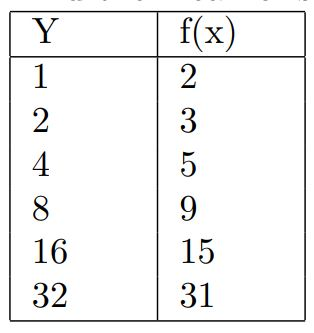Hint: Find the squared error for each prediction and take the mean of that.

• 1
• 2
• 1.5
• 0
`Answer :- a. 1`

7. Consider the following statements:

Statement A: In Forward stepwise selection, in each step, that variable is chosen which has the maximum correlation with the residual, then the residual is regressed on that variable, and it is added to the predictor.
Statement B: In Forward stagewise selection, the variables are added one by one to the previously selected variables to produce the best fit till then

• Both the statements are True.
• Statement A is True, and Statement B is False
• Statement A is False and Statement B is True
• Both the statements are False.
`Answer :- a. Both the statements are True.`

8. The linear regression model y=a0+a1x1+a2x2+…….+apxp is to be fitted to a set of N training data points having p attributes each. Let X be N×(p+1) vectors of input values (augmented by 1‘s), Y be N×1 vector of target values, and θθ be (p+1)×1 vector of parameter values (a0,a1,a2,…,ap. If the sum squared error is minimized for obtaining the optimal regression model, which of the following equation holds?

• XTX=XY
• Xθ=XT
• XTXθ =Y
• XTXθ=XTY
`Answer :- d. XTXθ=XTY`

9. Which of the following statements is true regarding Partial Least Squares (PLS) regression?

• PLS is a dimensionality reduction technique that maximizes the covariance between the predictors and the dependent variable.
• PLS is only applicable when there is no multicollinearity among the independent variables.
• PLS can handle situations where the number of predictors is larger than the number of observations.
• PLS estimates the regression coefficients by minimizing the residual sum of squares.
• PLS is based on the assumption of normally distributed residuals.
• All of the above.
• None of the above.
`Answer :- a`

10. Which of the following statements about principal components in Principal Component Regression (PCR) is true?

• Principal components are calculated based on the correlation matrix of the original predictors.
• The first principal component explains the largest proportion of the variation in the dependent variable.
• Principal components are linear combinations of the original predictors that are uncorrelated with each other.
• PCR selects the principal components with the highest p-values for inclusion in the regression model.
• PCR always results in a lower model complexity compared to ordinary least squares regression.
`Answer :- c. Principal components are linear combinations of the original predictors that are uncorrelated with each other.`

## NPTEL Introduction To Machine Learning Week 1 Assignment Answer 2023

1. Which of the following is a supervised learning problem?

• Grouping related documents from an unannotated corpus.
• Predicting credit approval based on historical data.
• Predicting if a new image has cat or dog based on the historical data of other images of cats and dogs, where you are supplied the information about which image is cat or dog.
• Fingerprint recognition of a particular person used in biometric attendance from the fingerprint data of various other people and that particular person.
`Answer :- b, c, d`

2. Which of the following are classification problems?

• Predict the runs a cricketer will score in a particular match.
• Predict which team will win a tournament.
• Predict whether it will rain today.
`Answer :- b, c, d`

3. Which of the following is a regression task?

• Predicting the monthly sales of a cloth store in rupees.
• Predicting if a user would like to listen to a newly released song or not based on historical data.
• Predicting the confirmation probability (in fraction) of your train ticket whose current status is waiting list based on historical data.
• Predicting if a patient has diabetes or not based on historical medical records.
• Predicting if a customer is satisfied or unsatisfied from the product purchased from ecommerce website using the the reviews he/she wrote for the purchased product.
`Answer :- a, c`

4. Which of the following is an unsupervised learning task?

• Group audio files based on language of the speakers.
• Group applicants to a university based on their nationality.
• Predict a student’s performance in the final exams.
• Predict the trajectory of a meteorite.
`Answer :- a, b`

5. Which of the following is a categorical feature?

• Number of rooms in a hostel.
• Gender of a person
• Your weekly expenditure in rupees.
• Ethnicity of a person
• Area (in sq. centimeter) of your laptop screen.
• The color of the curtains in your room.
• Number of legs an animal.
• Minimum RAM requirement (in GB) of a system to play a game like FIFA, DOTA.
`Answer :- b, d, f`

6. Which of the following is a reinforcement learning task?

• Learning to drive a cycle
• Learning to predict stock prices
• Learning to play chess
• Leaning to predict spam labels for e-mails
`Answer :- a, c`

7. Let X and Y be a uniformly distributed random variable over the interval [0,4][0,4] and [0,6][0,6] respectively. If X and Y are independent events, then compute the probability, P(max(X,Y)>3)

• 1/6
• 5/6
• 2/3
• 1/2
• 2/6
• 5/8
• None of the above
`Answer :- f (5/8)`

8. Find the mean of 0-1 loss for the given predictions: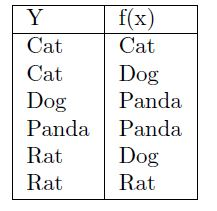• 1
• 0
• 1.5
• 0.5
`Answer :- d (0.5)`

9. Which of the following statements are true? Check all that apply.

• A model with more parameters is more prone to overfitting and typically has higher variance.
• If a learning algorithm is suffering from high bias, only adding more training examples may not improve the test error significantly.
• When debugging learning algorithms, it is useful to plot a learning curve to understand if there is a high bias or high variance problem.
• If a neural network has much lower training error than test error, then adding more layers will help bring the test error down because we can fit the test set better.
`Answer :- b, d`

10. Bias and variance are given by:

• E[f^(x)]−f(x),E[(E[f^(x)]−f^(x))2]
• E[f^(x)]−f(x),E[(E[f^(x)]−f^(x))]2
• (E[f^(x)]−f(x))2,E[(E[f^(x)]−f^(x))2]
• (E[f^(x)]−f(x))2,E[(E[f^(x)]−f^(x))]2
`Answer :- a`
Scroll to Top